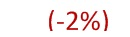QlikView Scripting

Discussion Board for collaboration on QlikView Scripting.

Highlighted
New Contributor III

Formating: Using fabs() and num() functions in text object

Dear fellow peers,

Table and charts formatting can done easily in 'number' tab, however when it comes to text object all expression has to be scripted for the desired result.

Has anyone encounter any issue using both function combination in a text object? It seem that both functions does not gel up mutually.

Current result is not showing absolute value:I am trying to achieve the conditions:

1. absolute value (using fabs)
2. percentage format (using num)
3. negative value with brackets using nested if statements.

Hence, my expressions might be a little heavier to read.

if(v%varhc<0,

'('&
//bracket
fabs(num((
IF(Dim2_ID='A',sum(Value),
IF(Dim2_ID='B',sum(Value2),
IF(Dim2_ID='C',sum(Value3),
IF(Dim2_ID='D',sum(Amount)))))
-(
sum( Amount)
))
/
IF(Dim2_ID='A',sum(Value),
IF(Dim2_ID='B',sum(Value2),
IF(Dim2_ID='C',sum(Value3),
IF(Dim2_ID='D',sum(Amount))))))
,'#,##0%')) //% format
&')',       //bracket

v%varhc)

**Note: v%varhc=IF(Dim2_ID='A',sum(Value),
IF(Dim2_ID='B',sum(Value2),
IF(Dim2_ID='C',sum(Value3),
IF(Dim2_ID='D',sum(Amount)))))
-(
sum( Amount)

Thanks for the advice.

Regards,

Nelson

Tags (5)
4 Replies
Honored Contributor III

Re: Formating: Using fabs() and num() functions in text object

Any reason for using '('&    //bracket,

couldn't understand use of this extra bracket with single quote in both the sides.

Honored Contributor III

Re: Formating: Using fabs() and num() functions in text object

Oh yeah,got it, you r clubbing final value for display purpose.

I just tried like this - =Num(fabs(-1/2),'#,##0.0%') it shows proper value but when I used fabs(Num - percentage didn't show up. It seems fabs is changing the format.

Can you try Num(Fabs) and see the result..Partner

Re: Formating: Using fabs() and num() functions in text object

The num() function can take a format as 'Format1;format2;format3' where Format1 is for positive values, Format 2 is for negative values and Format3 is for exact 0-values.

You might not need the fabs at all.

=num(-100,'# ##0%;(# ##0%)')

will display (100%)MVP & Luminary

Re: Formating: Using fabs() and num() functions in text object

Try using a couple of variables:

vField: pick(match(Dim2_ID, 'A','B','C','D'),'Value','Value2','Value3','Amount')

vSum: sum(\$(vField))

Expression: =num(1-\$(vSum)/sum(Amount),'# ##0%;(# ##0%)','.',' ')

talk is cheap, supply exceeds demand